Complete guide to crack Permutation and Combination: Permutation and combination are all about counting and arrangements made from a certain group of data. The meaning of both these terms is explained here in this article, along with formulas and examples. This is one of the most important topics in the list of mathematics.

## Permutation And Combination Tricks For Banks Aptitude Exams

Dear IBPS PO Candidates

After reading our Tips to Crack Quantitative Aptitude Section IBPS PO Prelim, many of our readers requested tips on the toughest and onerous section of Quantitative Aptitude – Permutation and Combination. There have been countless instances where some candidates lost valuable time solving just one question from this topic while others couldn’t get the logic right, costing them their selection in IBPS PO Prelims.

So how do you become a master of Permutation and Combination? The tried and tested way is to get your basics right, solve as many questions as you can and voila you become the master. But considering that IBPS PO Prelim is happening this weekend, there is not much time to become a master. So We have prepared a list of tips, tricks and things to remember for helping you crack Permutation and Combination in less time.

BASICS

We will start by getting the basics right. After all, you need a solid foundation if you are to construct a skyscraper.

Permutation vs Combination

Ever got confused between which one is what? I did. So here’s the basic difference between the two:

A combination means selecting things at random (order doesn’t matter) while Permutation means the arrangement of objects in a particular order.

For example, from all the subjects and sections that can be asked in an exam, you have to study combination  of 3 sections for IBPS PO Prelims : Quant, Reasoning and Verbal. Here it doesn’t makes any difference if i write the above sections as reasoning , verbal and quant. The sections to attempt will remain same.

The order in which you will attempt the section, however will be different. Some people will attempt in the order of verbal, quant and reasoning. Some others may want to attempt in the order – verbal, reasoning and quant. Here the order does matter. So the total ways in which someone can attempt the complete paper (3x2x1 = 6)  is known as permutation.

A simpler way to remember is :

Permutation sounds complex. Well it is because in permutation each and every detail matters.

Combination is simpler and easy: Details don’t matter.

Permutations is a tool to make lists where order matters whereas combinations is used for groups where order doesn’t matters.

With the difference clear between the two, it time to go deeper into ocean of the permutations and combinations.

Factorial:

Let n be a positive integer. Then n factorial (n!) can be defined as

n! = n(n-1)(n-2)…1

5! = 5 x 4 x 3 x 2 x 1

Special Cases

0! = 1

1! = 1

Permutations:

Suppose, there is a swimming competition going on. We have 8 participants: A, B, C, D, E, F, G and H.

We have to award Gold, Silver and Bronze medals to the top 3 contestants respectively.

In how many ways can award the medals to the 8 participants.

We will use permutations since the order in which we have to distribute the medals matters.

• To give away the gold medal we have: 8 choices: A B C D E F G H. Assume A wins the Gold.
• Now for the Silver medal we are left with 7 choices(since A already won the gold medal): B C D E F G H. Assume B wins the silver.
• With A and B already bagging gold and silver we are left with 6 choices for the Bronze medal:  C D E F G H. Let’s assume C wins the bronze.

Note, we had 8 choices at first, then 7, then 6. Hence, the total number of ways was 8 * 7 * 6 = 336.

Digging deeper into the details , we have to arrange 3 people out of 8 competitors. To achieve this, we started with all the options (8) then took them away one at a time (7, then 6) until there were no medals left.

A factorial of a number is obtained by multiplying all the natural numbers starting from 1 to the number. So factorial 8 will be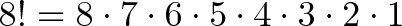$\displaystyle{8! = 8 \cdot 7 \cdot 6 \cdot 5 \cdot 4 \cdot 3 \cdot 2 \cdot 1 }$ .( Factorial of a number n is denoted by n!)

But we need only 8 x 7 x 6 for our answer.

We need to remove 5 x x 3 x x 1. Notice it is the expression for 5!.

So, if we do 8!/5! we get: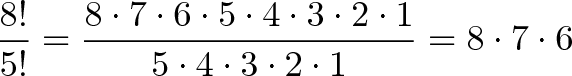$\displaystyle{\frac{8!}{5!} = \frac{8 \cdot 7 \cdot 6 \cdot 5 \cdot 4 \cdot 3 \cdot 2 \cdot 1}{5 \cdot 4 \cdot 3 \cdot 2 \cdot 1} = 8 \cdot 7 \cdot 6}$

You must be wondering why we took the number 5? Because, 5 people were left after we awarded 3 medals from 8. i.e.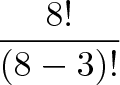$\displaystyle{\frac{8!}{(8-3)!}}$

where 8!/(8-3)! “Use the first 3 out of 8!”.

So, If we have items total and want to pick k in a particular order, we get: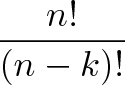$\displaystyle{\frac{n!}{(n-k)!}}$

This is the permutation formula: From a total of n items, you need to find number of ways in which k items can be ordered: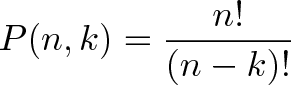$\displaystyle{P(n,k) = \frac{n!}{(n-k)!}}$

COMBINATIONS

Combinations are simpler as order doesn’t matter. Let us assume in the above swimming contest,  instead of medals I am to distribute one pencil each to the first 3 winners.

In how many ways, can you distribute 3 pencils to 8 people?

In this case the order we pick people don’t matter. If I give a pencil first to A, then to B and then to C, it’s same as giving it to C first, followed by A and B.

So, if we have 3 pencils to distribute, there are 3! or 6 variations for every choice we pick. If we want to know how many combinations we have, calculate all the permutations and divide them by all the redundancies. In our case, we have 336 (=8 x 7 x 6) permutations and we divide it by the 6 redundancies for each permutation to get 336/6 = 56.

The general formula is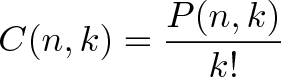$\displaystyle{C(n,k) = \frac{P(n,k)}{k!}}$

which means “Find all the ways to pick k people from n, and divide by the k! variants”. So the combination formula, or the number of ways to combine k items from a set of n is:$\displaystyle{C(n,k) = \frac{n!}{(n-k)!k!}}$

There are always fewer combinations than permutations.

The Multiplication Theorem:

If a task can be performed in x different ways,  following which a second task can be performed in y different ways, then the two operations in succession can be performed in x × y different ways. For example:

Our cricket team has 3 shirts and 4 pants, how many different kits can be made.

For the shirt we have 3 choices. Similarly for pants we have 4 choices. Just multiply the choices to get the answer. That is 3 x 4 = 12. With first shirt you can choose one from the 4 pants. With second shirt you again can choose any one from the 4 pants and so on.

If a task can be performed in different ways and another independent operation can be performed in y different ways, either of the two operations can be performed in (x+y) ways.

Repetition :

You have to arrange n things and you have n choices everytime.

(In other words, there are n possibilities for the first choice, THEN there are n possibilites for the second choice, and so on, multplying each time.) If we have to put in r places

n × n × … (r times) = nr

Suppose we have a number lock with 3 dials. Here the number of ways you can arrange 10 digits (0 1 2 3 4 5 6 7 8 9) in 3 dials will be

= 103

This is because the correct combination can have same number in all the 3 dials. So repetition is allowed.

Don’t assume repetition unless mentioned in the question.

Solving a permutation or combination problem is a two step process,

1. Recognizing the problem type: permutation or combination.

2. Using formulas or models to count the possibilities.

The problem students face is how to identify whether the problem is for permutation or for combination?

The best way to do is switch the items of the question and checking is the result the same?

If you need to pick a Captain and Vice-captain, then switching them changes the result. So, picking a Captain and Vice-captain from a set of candidates is permutation.

If you want to choose 2 players for a fight, then switching the order doesn’t change the result: either way, they are fighting each other. Hence, it’s a combination.

The second question to ask is whether repetition is allowed or not.

Formula and Shortcuts

• Number of permutations of n different things, taken r at a time, when a particular thing is to be always included in each arrangement is:  rn−1Pr−1
•  Number of permutations of n different things, taken r at a time, when a particular thing is never taken in each arrangement is: n−1Pr
•  Number of permutations of n different things, taken all at a time, when m specified things always come together is: m!×(n−m+1)!
• Number of permutations of n different things, taken all at a time, when m specified never come together is:     n!−[m!×(n−m+1)!]
• The number of permutations of n dissimilar things taken r at a time when k(< r) particular things always occur is: [nkPrk]×[rPk]
• The number of permutations of n dissimilar things taken r at a time when k particular things never occur is:     nkPr
• The number of permutations of n dissimilar things taken r at a time when repetition of things is allowed any number of times is: nr
• The number of permutations of n different things, taken not more than r at a time, when each thing may occur any number of times is: n+n2+n3+….+nr=n(nr−1) / n−1
• The number of permutations of n different things taken not more than r at a time:
nP1+nP2+nP3+…+nPr
• n!=n×(n1)!
• (n+1).nPr=n+1Pr+1
• nCr=nC n−r
• n−1Cr−1+n−1Cr=nCr
• 0!=1!=
•$\displaystyle{P(n,k) = \frac{n!}{(n-k)!}}$
•$\displaystyle{C(n,k) = \frac{n!}{(n-k)!k!}}$

Now apply the knowledge on the following Permutation and Combination questions handpicked from IBPS PO papers.

1. Consider the palindrome RADAR. Whichever way you read it, from left to right or from right to left, you get the same word. Find the maximum possible number of 5-letter palindromes.

2. How many 4-digit numbers can be formed with the digits 1,2,5,6 and 9 if the digits are not repeated?

3. Suppose you can travel from a place A to a place B by 2 buses, from place B to place C by 3 buses, from place C to place D by 4 buses and from place D to place E by 1 buses. In how many ways can you travel from A to E?

4.  7 students (A, B, C, D, E, F and G)are staying in a hall in a hostel and they are allotted 7 beds. Among them, A does not want a bed next to B because B snores. Then, in how many ways can you allot the beds?

5. In how many ways can letters of the word ‘ALPHABET’ be arranged such that vowels an

i) All vowels are together.

ii) Vowels are never together.

6. From 4 consonants and 3 vowels, how many words can be formed using 3 consonants and 2 vowels?

7. A committee of 5 persons is to be formed from 6 men and 4 women. In how many ways can this be done when:

(i) At least 2 women are included?

(ii) At most 2 women are included?

8. In a box, there are 5 black pens, 3 white pens and 4 red pens. In how many ways can 2 black pens, 2 white pens and 2 red pens can be chosen?

9. In how many different ways can the letters of the word ‘MACHINE’ be arranged so that the vowels may occupy only the odd positions?

10. Find the number of ways in which 5 different beads can be arranged to form a necklace.

Try to answer these questions and comment them below.

We will post video solutions and answers to these questions tomorrow, so make sure to check our bulletin again

Video Solutions to selected problems

1.bhaupander kumar

hello sir,
IBPS PO परीक्षा की तैयारी के लिए आप की टिप्स सभी विद्यार्थियों के लिए राम बाण के समान है जिसके लिए आप का बहुत धन्यबाद।

sir ji अगर आप SSC 10+2 परीक्षा से सम्बंधित कुछ मार्गदर्शन करदें तो आप की अति किर्पया होगी।

धन्यबाद……

2.Vaibhav

Sure Kshitij.
We will soon provide PDF. Will notify you

3. Sir itni useful information plz plz plz pdf m provided kiya kijiye.
n infact provide all these guides in pdf format.
So dat we can read n study it anytime we want. we can revise it as well. N also we can share it to our frnds as you always say ‘SHARING IS CARING’
Thank you ? sir

4. Haan hai…

5.rakhi

Hi Sir,
aapke post mere liye bhut usefull hai.
sir ibps po preliminary me negative marking hai ya nai.plz sir reply.